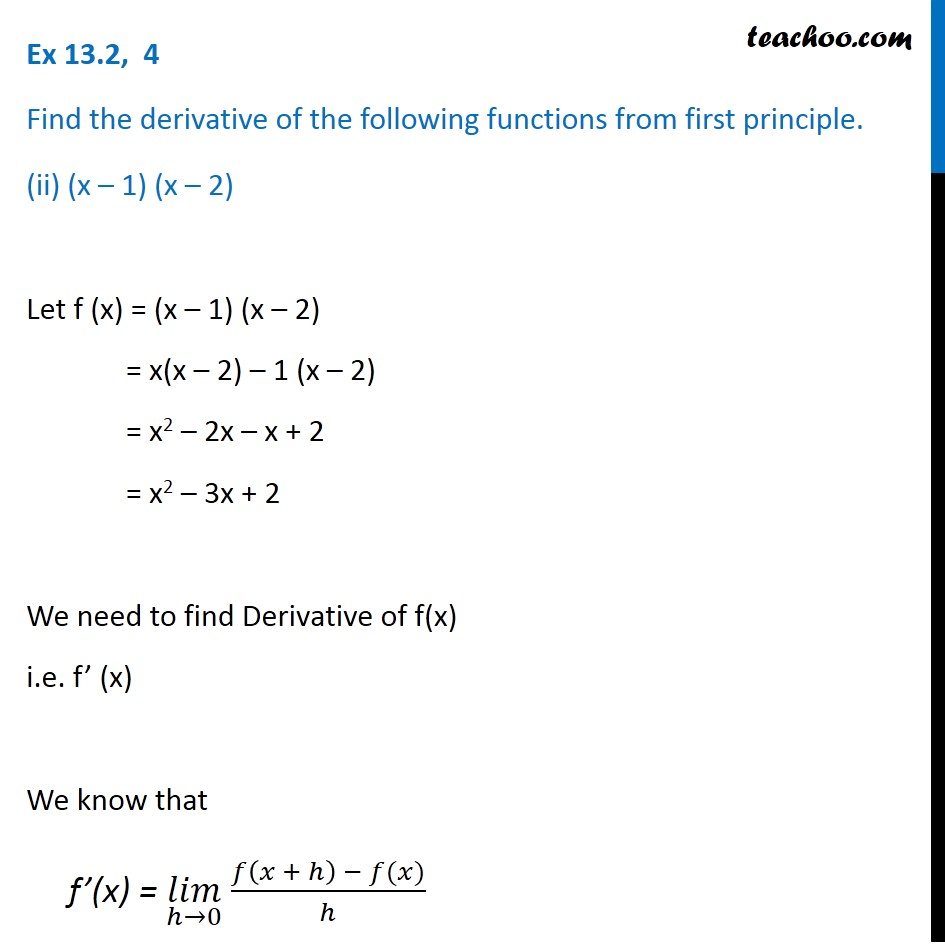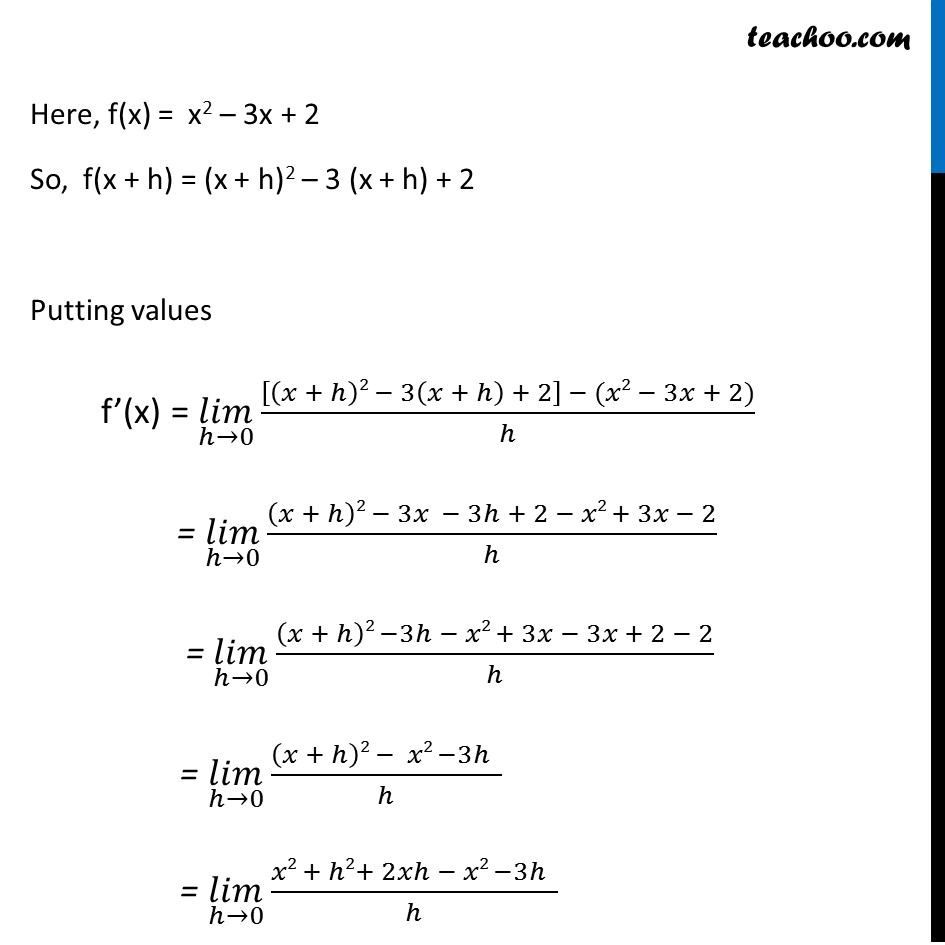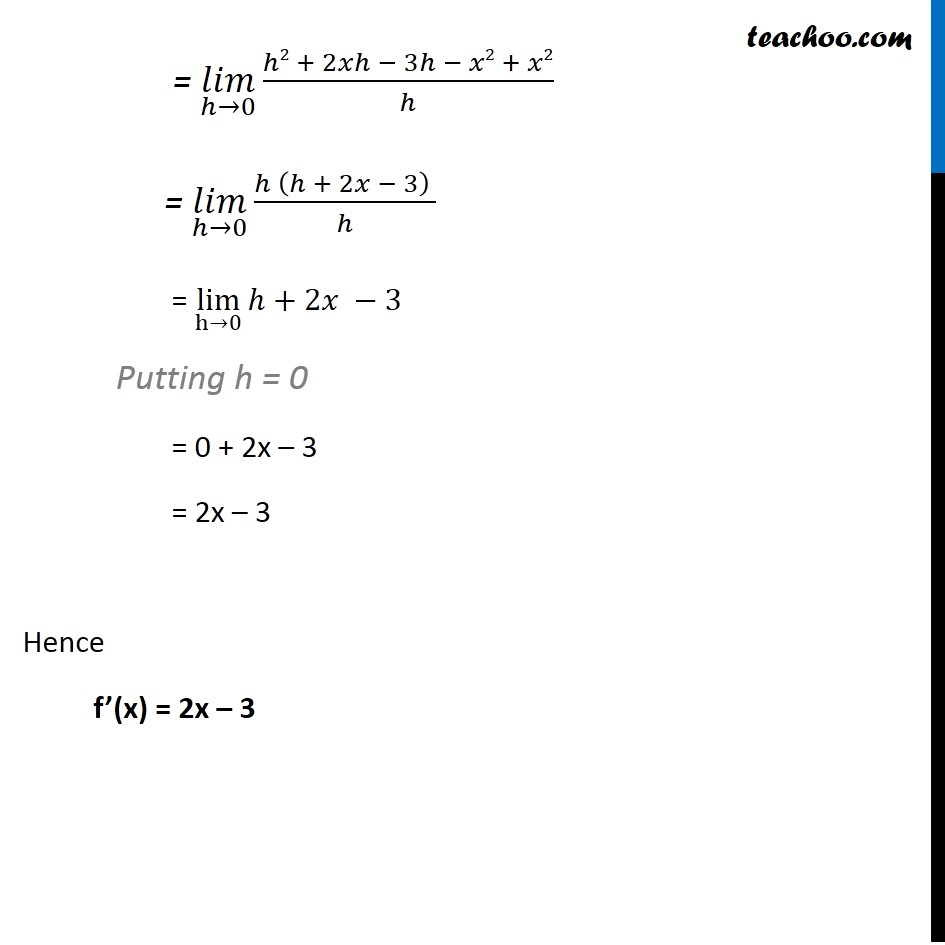Ex 13.2

Chapter 13 Class 11 Limits and Derivatives
Serial order wiseGet live Maths 1-on-1 Classs - Class 6 to 12

### Transcript

Ex 13.2, 4 Find the derivative of the following functions from first principle. (ii) (x – 1) (x – 2) Let f (x) = (x – 1) (x – 2) = x(x – 2) – 1 (x – 2) = x2 – 2x – x + 2 = x2 – 3x + 2 We need to find Derivative of f(x) i.e. f’ (x) We know that f’(x) = (𝑙𝑖𝑚)┬(ℎ→0) 𝑓⁡〖(𝑥 + ℎ) − 𝑓(𝑥)〗/ℎ Here, f(x) = x2 – 3x + 2 So, f(x + h) = (x + h)2 – 3 (x + h) + 2 Putting values f’(x) = (𝑙𝑖𝑚)┬(ℎ→0)⁡〖([(𝑥 + ℎ)2 − 3(𝑥 + ℎ) + 2] − (𝑥2 − 3 + 2))/ℎ〗 = (𝑙𝑖𝑚)┬(ℎ→0)⁡〖((𝑥 + ℎ)2 − 3𝑥 − 3ℎ + 2 − 𝑥2 + 3𝑥 − 2)/ℎ〗 = (𝑙𝑖𝑚)┬(ℎ→0)⁡〖((𝑥 + ℎ)2 −3ℎ − 𝑥2 + 3𝑥 − 3𝑥 + 2 − 2)/ℎ〗 = (𝑙𝑖𝑚)┬(ℎ→0)⁡〖((𝑥 + ℎ)2 − 𝑥2 −3ℎ )/ℎ〗 = (𝑙𝑖𝑚)┬(ℎ→0)⁡〖(𝑥2 + ℎ2+ 2𝑥ℎ − 𝑥2 −3ℎ )/ℎ〗 = (𝑙𝑖𝑚)┬(ℎ→0)⁡〖(ℎ2 + 2𝑥ℎ − 3ℎ − 𝑥2 + 𝑥2)/ℎ〗 = (𝑙𝑖𝑚)┬(ℎ→0)⁡〖(ℎ (ℎ + 2𝑥 − 3) )/ℎ〗 = lim┬(h→0)⁡〖ℎ+2𝑥 −3〗 Putting h = 0 = 0 + 2x – 3 = 2x – 3 Hence f’(x) = 2x – 3